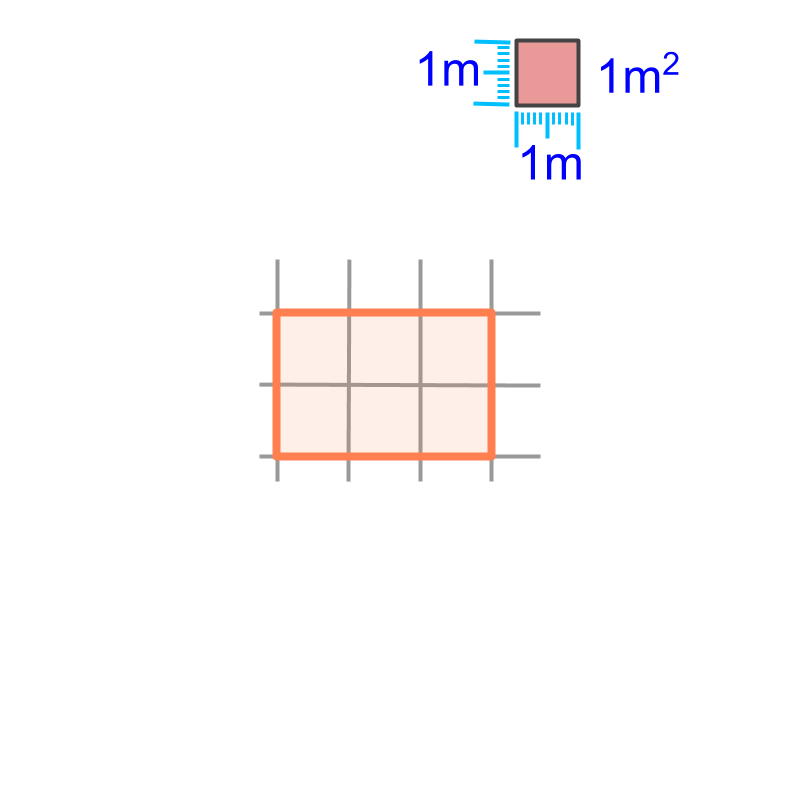maths > mensuration-basics

Introduction to Measuring Area

what you'll learn...

Overview

Area of a plane figure : The surface-span of a plane figure is the area of the surface. It is measured in square meter (or in one of other derived or similar forms).Area is specified as a number in reference to the surface-span of a square of $1$$1$ meter side.

surface-span

The length of a rod is the distance-span measured in meter.

For a surface of some shape (like a paper, or a leaf), the measure of the surface-span is area. Area is the surface-span of an enclosed 2D-region.

To specify distance-span or length, a reference-prototype-standard (ie a metal rod of specific material at specific temperature) was defined. Any length measurement is specified in reference to the prototype-standard.

Similarly, to specify area, a reference-prototype-standard can be defined. But, it is simpler to define the measurement of area using the already defined measure of length.To specify the surface-span or area of a surface, a square of side $1$$1$ meter is taken as the reference. The area of that is one square meter or $1{m}^{2}$$1 {m}^{2}$. In the figure this is shown in the top right corner.

Area of a surface is given in reference to the area of a square of side $1$$1$ meter. In the figure, the area of the given rectangle is the number of $1$$1$ square meter squares fit in that rectangle. It is counted to $6$$6$, so the area of the rectangle is $6{m}^{2}$$6 {m}^{2}$.

The statement "area of the paper is $3$$3$ square meter" specifies that "The surface-span of the paper equals $3$$3$ surface-spans of a square of side $1$$1$ meter".

summary

Area of a plane figure : The surface-span of a plane figure is the area of the surface. It is measured in square meter (or in one of other derived or similar forms).Area is specified as a number in reference to the surface-span of a square of $1$$1$ meter side.

Outline

The outline of material to learn "Mensuration basics : Length, Area, & Volume" is as follows.

•  Measuring Basics

→   Introduction to Standards

→   Measuring Length

→   Accurate & Approximate Meaures

→   Measuring Area

→   Measuring Volume

→   Conversion between Units of Measure

•  2D shapes

→   Perimeter of Polygons

→   Area of Square & rectangle

→   Area of Triangle

→   Area of Polygons

→   Perimeter and area of a Circle

→   Perimeter & Area of Quadrilaterals

•  3D shapes

→   Surface Area of Cube, Cuboid, Cylinder

→   Volume of Cube, Cuboid, Cylinder